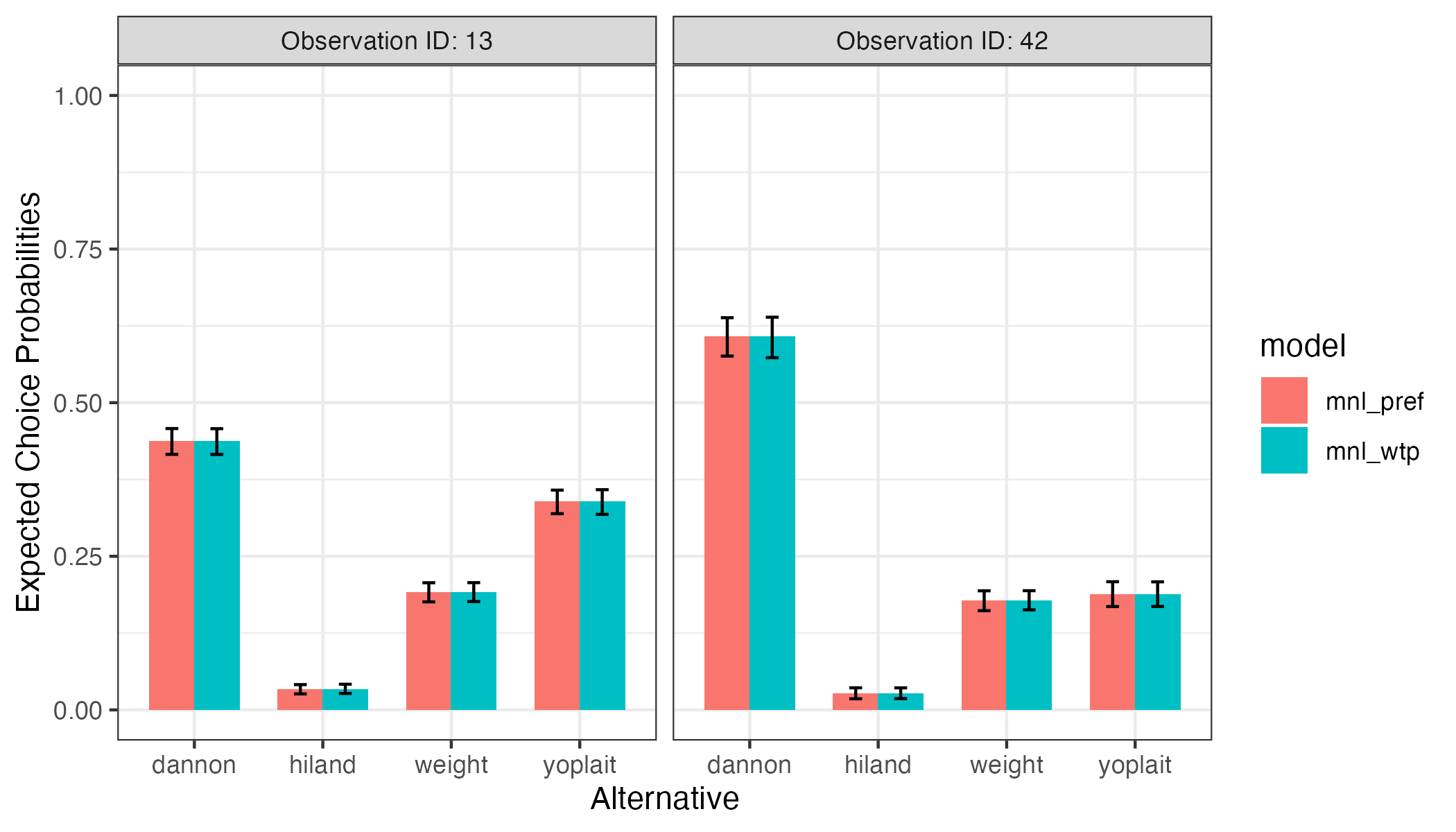Once a model has been estimated, it can be used to predict the expected choice probabilities for a set of alternatives. This vignette demonstrates examples of how to so using the predictProbs() function along with the results of an estimated model.

# The data

To predict choice probabilities, you first need to create a set of alternatives where each row is an alternative and each column an attribute. In this example, I use two of the choice observations from the yogurt dataset (note that if you include more than one set of alternatives you also have to specify an obsID):

alts <- subset(
yogurt, obsID %in% c(42, 13),
select = c('obsID', 'alt', 'price', 'feat', 'brand'))

alts
#>     obsID alt price feat   brand
#> 49     13   1   8.1    0  dannon
#> 50     13   2   5.0    0  hiland
#> 51     13   3   8.6    0  weight
#> 52     13   4  10.8    0 yoplait
#> 165    42   1   6.3    0  dannon
#> 166    42   2   6.1    1  hiland
#> 167    42   3   7.9    0  weight
#> 168    42   4  11.5    0 yoplait

# Predicting choice probabilities with multinomial logit models

## Preference space parameterization

In the example below, I estimate a preference space MNL model called mnl_pref. I can then use the predictProbs() function with the mnl_pref model to predict the choice probabilities for each set of alternatives in the alts data frame:

# Estimate the model
mnl_pref <- logitr(
data   = yogurt,
choice = 'choice',
obsID  = 'obsID',
pars   = c('price', 'feat', 'brand')
)

# Predict choice probabilities
probs_mnl_pref <- predictProbs(
model = mnl_pref,
alts  = alts,
altID = "alt",
obsID = "obsID"
)
probs_mnl_pref
#>   obsID alt  prob_mean   prob_low  prob_high
#> 1    13   1 0.43685145 0.41551636 0.45767025
#> 2    13   2 0.03312986 0.02634065 0.04197329
#> 3    13   3 0.19155548 0.17638379 0.20740073
#> 4    13   4 0.33846321 0.31883538 0.35819210
#> 5    42   1 0.60764778 0.57298037 0.63952161
#> 6    42   2 0.02602007 0.01844817 0.03666175
#> 7    42   3 0.17803313 0.16254368 0.19433957
#> 8    42   4 0.18829902 0.16820048 0.20962336

The results show the expected choice probabilities for each alternative. The low and high values show a 95% confidence interval estimated using the Krinsky and Robb parametric bootstrapping method (Krinsky and Robb 1986). You can change the CI level with the optional ci argument (e.g. a 90% CI is obtained with ci = 0.90).

## WTP space parameterization

You can also use WTP space models to predict choice probabilities. For example, here are the results from an equivalent model but in the WTP space:

# Estimate the model
mnl_wtp <- logitr(
data       = yogurt,
choice     = 'choice',
obsID      = 'obsID',
pars       = c('feat', 'brand'),
price      = 'price',
modelSpace = 'wtp',
numMultiStarts = 10
)

# Predict choice probabilities
probs_mnl_wtp <- predictProbs(
model = mnl_wtp,
alts  = alts,
altID = "alt",
obsID = "obsID"
)
#> NOTE: Using results from run 8 of 10 multistart runs
#> (the run with the largest log-likelihood value)
probs_mnl_wtp
#>   obsID alt  prob_mean   prob_low  prob_high
#> 1    13   1 0.43686118 0.41520035 0.45757324
#> 2    13   2 0.03312960 0.02645117 0.04218890
#> 3    13   3 0.19154819 0.17624652 0.20798421
#> 4    13   4 0.33846102 0.31879172 0.35891319
#> 5    42   1 0.60767142 0.57242148 0.63901654
#> 6    42   2 0.02601792 0.01836527 0.03669751
#> 7    42   3 0.17802356 0.16234301 0.19508236
#> 8    42   4 0.18828710 0.16823284 0.20918393

Since these two models are equivalent except in different spaces, the predicted choice probabilities should be the same.

# Predicting probabilities with mixed logit models

## Preference space parameterization

You can also use mixed logit models to predict choice probabilities. Heterogeneity is modeled by simulating draws from the population estimates of the estimated model. Here is an example using a preference space mixed logit model:

# Estimate the model
mxl_pref <- logitr(
data     = yogurt,
choice   = 'choice',
obsID    = 'obsID',
pars     = c('price', 'feat', 'brand'),
randPars = c(feat = 'n', brand = 'n'),
numMultiStarts = 5
)

# Predict choice probabilities
probs_mxl_pref <- predictProbs(
model = mxl_pref,
alts  = alts,
altID = "alt",
obsID = "obsID"
)
probs_mxl_pref
#>   obsID alt  prob_mean   prob_low  prob_high
#> 1    13   1 0.45219073 0.39712730 0.47081159
#> 2    13   2 0.02780546 0.02272863 0.08881051
#> 3    13   3 0.21019800 0.18393940 0.22900610
#> 4    13   4 0.30980580 0.27932247 0.36616376
#> 5    42   1 0.60383358 0.50122199 0.64836191
#> 6    42   2 0.06797921 0.02776386 0.14701828
#> 7    42   3 0.18721068 0.16160022 0.20758873
#> 8    42   4 0.14097653 0.11411015 0.22517057

## WTP space parameterization

Likewise, mixed logit WTP space models can also be used to predict choice probabilities:

# Estimate the model
mxl_wtp <- logitr(
data       = yogurt,
choice     = 'choice',
obsID      = 'obsID',
pars       = c('feat', 'brand'),
price      = 'price',
randPars   = c(feat = 'n', brand = 'n'),
modelSpace = 'wtp',
numMultiStarts = 5
)

# Predict choice probabilities
probs_mxl_wtp <- predictProbs(
model = mxl_wtp,
alts  = alts,
altID = "alt",
obsID = "obsID"
)
probs_mxl_wtp
#>   obsID alt  prob_mean   prob_low  prob_high
#> 1    13   1 0.45134184 0.40081021 0.46968446
#> 2    13   2 0.02782395 0.02301138 0.09224299
#> 3    13   3 0.20985172 0.18556229 0.22940154
#> 4    13   4 0.31098250 0.27875473 0.35998801
#> 5    42   1 0.60310825 0.52405495 0.63738901
#> 6    42   2 0.06830282 0.02751463 0.15554164
#> 7    42   3 0.18706005 0.16145448 0.20805114
#> 8    42   4 0.14152888 0.10857708 0.21589030

# Compare results

Here is a bar chart comparing the predicted choice probabilities from each model:

library(ggplot2)
library(dplyr)

probs <- rbind(
probs_mnl_pref, probs_mnl_wtp, probs_mxl_pref, probs_mxl_wtp) %>%
mutate(
model = c(rep("mnl_pref", 8), rep("mnl_wtp", 8),
rep("mxl_pref", 8), rep("mxl_wtp", 8)),
alt = rep(c("dannon", "hiland", "weight", "yoplait"), 8),
obs = paste0("Observation ID: ", obsID)
)

ggplot(probs, aes(x = alt, y = prob_mean, fill = model)) +
geom_bar(stat = 'identity', width = 0.7, position = "dodge") +
geom_errorbar(aes(ymin = prob_low, ymax = prob_high),
width = 0.2, position = position_dodge(width = 0.7)) +
facet_wrap(vars(obs)) +
scale_y_continuous(limits = c(0, 1)) +
labs(x = 'Alternative', y = 'Expected Choice Probabilities') +
theme_bw()